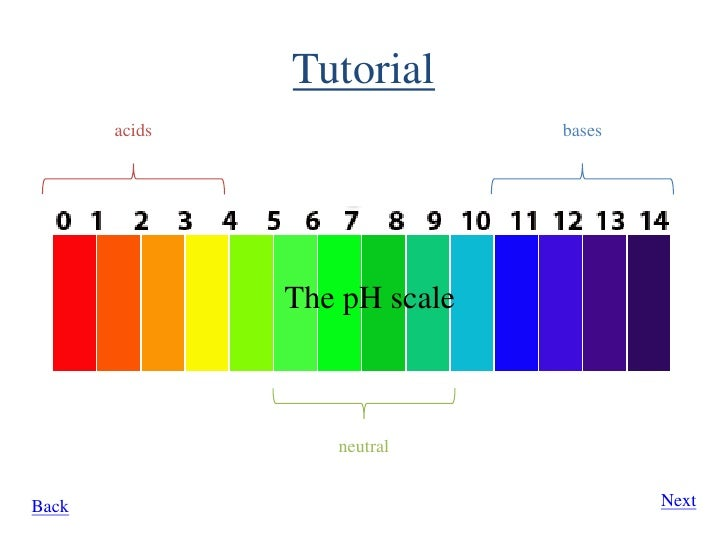# When is an aqueous solution basic? What pH?

Apr 12, 2016

It is basic at ${25}^{\circ} \text{C}$ and $\text{1 atm}$, when pH is greater than 7.

#### Explanation:

For any aqueous solution at ${25}^{\circ} \text{C}$ and $\text{1 atm}$:

• $\text{pH" = 7 rightarrow "neutral}$
• $\text{pH" < 7 rightarrow "acidic}$
• $\text{pH" > 7 rightarrow "basic}$

We may note that pH can take any value (even negative values or equal to zero).

P.S. I do not have too much time, but I strongly recommend you to take a look at the definition of pH as the logarithm of $\text{H"^{+}}$ concentration, i.e. "pH" = -log["H"^(+)].

Apr 23, 2016

Basic

#### Explanation:

The higher the pH number is, the more alkaline (or basic) it gets.
Neutral is 7 so anything higher than 7 is alkaline and anything lower than 7 is acidic.